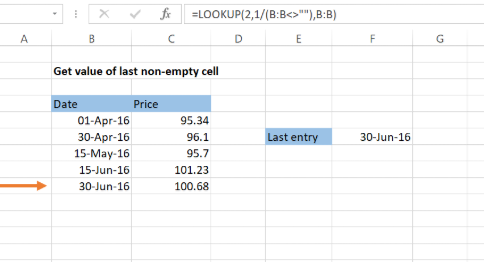Get instant live expert help with Excel or Google Sheets“My Excelchat expert helped me in less than 20 minutes, saving me what would have been 5 hours of work!”

#### Post your problem and you'll get expert help in seconds

Your message must be at least 40 characters
Our professional experts are available now. Your privacy is guaranteed.

# Get the Value of the Last Non-empty Cell in Excel

Have you been searching around for a clean and effective way to get the value of the last non-empty cell in Excel? Fortunately, there is a simple way to do this using the LOOKUP function. Here is an explanation as well as an example.

## Find value of the last non-empty cell

### Formula using LOOKUP

`=LOOKUP(2,1/(B:B<>""),B:B)`

### Explanation of formula

This non-array and the non-volatile formula are great to use the lookup function and find out the value of the last non-empty cell in a row or column. Here is how you can apply this formula to a situation.

### Example

Using B:B<>”” a particular array of TRUE and FALSE values is set up and then the lookup value is found.Figure 1. Get value of last non-empty cell by LOOKUP formula

We used the following formula in the above-mentioned example. The original formula modified to this form as it removes any errors that might be there in the last non-empty cell. ISBLANK is used with NOT to work around the errors which exist in there.

`=LOOKUP(2,1/(NOT(ISBLANK(B:B))),B:B)`

We added the ISNUMBER function than to get the last numeric value, and the resulting formula was like this:

`=LOOKUP(2,1/(ISNUMBER(B1:B100)),B1:B100)`

Then the following formula is used to find out the position (i.e., the row number or the column name) of the last non-empty cell.

`=LOOKUP(2,1/(B:B<>""),ROW(B:B))`

This way we find out the position of the row or column number/name of the last non-empty cell by feeding the corresponding row/column number/name in the lookup function. So, follow the instructions carefully and perform step by step in Excel to get the value of the last non-empty cell.

Still need some help with Excel formatting or have other questions about Excel? Connect with a live Excel expert here for some 1 on 1 help. Your first session is always free.

### Did this post not answer your question? Get a solution from connecting with the expert.Another blog reader asked this question today on Excelchat:## Subscribe to Excelchat.coAnother blog reader asked this question today on Excelchat: